# 5 Photoionization Modeling

We next proceed with more detailed photoionization analysis using the code XSTAR  (v2.2; Kallman & Bautista, 2001; Kallman et al., 1996; Kallman et al., 2004; Kallman et al., 2009), primarily developed for X-ray astronomy. This code solves the radiative transfer of ionizing radiation in a spherical gas cloud under a variety of physical conditions for astrophysically abundant elements, calculates ionization state and thermal balance, and produces the level populations, ionization structure, thermal structure, emissivity and opacity of a gas with specified density and composition, including the rates for line emission and absorption from bound-bound and bound-free transitions in ions. It utilizes the atomic database of Bautista & Kallman (2001), containing a large quantity of atomic energy levels, atomic cross section, recombination rate coefficients, transition probabilities, and excitation rates.

We assumed a spherical geometry with a covering fraction of, which is typical of the ionized absorbers in AGNs (Tombesi et al., 2010). Fundamental parameters in photoionization modeling are the total gas number density(in cm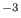), the total hydrogen column density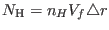(in cm), the ionization parameter(in ergcms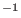; Tarter et al., 1969), and the turbulent velocity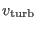(kms), where(in ergs) is the ionizing luminosity between 13.6 eV and 13.6 keV (i.e. 1 and 1000 Ryd),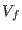is the volume filling factor of the ionized gas, and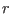andare the distance from the ionizing source and the thickness of the ionized gaseous shell (in units of cm), respectively. We set the initial gas temperature to the typical value ofK (Nicastro et al., 1999; Bianchi et al., 2005), and allowed the code to calculate it based on the thermal equilibrium of gas. We generated grids of XSTAR models (see §5.2) using an ionizing spectral energy distribution (SED) described in §5.1 (see also Figure4).Subsections
Ashkbiz Danehkar
2018-03-28